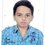# INMO 2017 Practice SET-II (Algebra only)

Hello friends, try these problems and post solutions :

$(1)$ For each positive integer $n$, show that there exists a positive integer $k$ such that

$k = f(x){ (x + 1) }^{ 2n }+ g(x)({ x }^{ 2n }+ 1)$

for some polynomials $f$, $g$ with integer coefficients, and find the smallest such $k$ as a function of $n$.

($2)$ Prove that there exists a polynomial $P(x, y)$ with real coefficients such that $P(x, y) \ge 0$ for all real numbers $x$ and $y$, which cannot be written as the sum of squares of polynomials with real coefficients.

$(3)$ Let ${ x }_{ 1 } = 2$ and ${ x }_{ n + 1 } = { x }^{ 2 }n - { x }_{ n } + 1$ for $n\ge 1$.

Prove that

$\large\ 1 - \frac { 1 }{ { { 2 }^{ 2 } }^{ n - 1 } } < \frac { 1 }{ { x }_{ 1 } } + \frac { 1 }{ { x }_{ 2 } } +...+ \frac { 1 }{ { x }_{ n } } < 1 - \frac { 1 }{ { { 2 }^{ 2 } }^{ n } }$.

$(4)$ Find, with proof, all nonzero polynomials $f(z)$ such that $f({ z }^{ 2 }) + f(z)f(z + 1) = 0$.

$(5)$ Find the least real number $r$ such that for each triangle with side lengths $a$, $b$, $c$,

$\large\ \frac { max(a, b, c) }{ \sqrt [ 3 ]{ { a }^{ 3 } + { b }^{ 3 } + { c }^{ 3 } + 3abc } } < r$.

$(6)$ Let $a$, $b$, $c$ be positive real numbers. Prove that

$\large\ { \left( \sum { \frac { a }{ b+c } } \right) }^{ 2016 } \le \left( \sum { \frac { { a }^{ 2016 } }{ { b }^{ 2016 } + { b }^{ 2015 }c } } \right) { \left( \sum { \frac { a }{ c + a } } \right) }^{ 2015 }$.Note by Priyanshu Mishra
4 years, 1 month ago

This discussion board is a place to discuss our Daily Challenges and the math and science related to those challenges. Explanations are more than just a solution — they should explain the steps and thinking strategies that you used to obtain the solution. Comments should further the discussion of math and science.

When posting on Brilliant:

• Use the emojis to react to an explanation, whether you're congratulating a job well done , or just really confused .
• Ask specific questions about the challenge or the steps in somebody's explanation. Well-posed questions can add a lot to the discussion, but posting "I don't understand!" doesn't help anyone.
• Try to contribute something new to the discussion, whether it is an extension, generalization or other idea related to the challenge.

MarkdownAppears as
*italics* or _italics_ italics
**bold** or __bold__ bold
- bulleted- list
• bulleted
• list
1. numbered2. list
1. numbered
2. list
Note: you must add a full line of space before and after lists for them to show up correctly
paragraph 1paragraph 2

paragraph 1

paragraph 2

[example link](https://brilliant.org)example link
> This is a quote
This is a quote
    # I indented these lines
# 4 spaces, and now they show
# up as a code block.

print "hello world"
# I indented these lines
# 4 spaces, and now they show
# up as a code block.

print "hello world"
MathAppears as
Remember to wrap math in $$ ... $$ or $ ... $ to ensure proper formatting.
2 \times 3 $2 \times 3$
2^{34} $2^{34}$
a_{i-1} $a_{i-1}$
\frac{2}{3} $\frac{2}{3}$
\sqrt{2} $\sqrt{2}$
\sum_{i=1}^3 $\sum_{i=1}^3$
\sin \theta $\sin \theta$
\boxed{123} $\boxed{123}$

Sort by:

I have solution of Q5 Is answer (-1)^2k +1 {( z(z-1)}^n

- 1 year, 8 months ago

I suppose that 6 uses the chebyshev's once

- 4 years, 1 month ago

This is the proposal so I don't know the answer.

- 4 years, 1 month ago

Not started yet. But i ve no idea of the first one pls post the sol or give a hint

- 4 years, 1 month ago

I cant understand what u say

- 4 years, 1 month ago

I just got the 3 one.its as follows prove by induction that the given expressions equals1-( (x1x2.......xn)^-1) then make the reqd changes in the inequality. By the way the above induction also showds that xn=(x1x2.......xn-1)+1 Now again induction on the transformed ineq completes the sol

- 4 years, 1 month ago

Nice, concise solution.

HAVE you solved the problem 6?

- 4 years, 1 month ago

Will the other discussions also be present on Brilliant Discussion link as this one

- 4 years, 1 month ago

Yes.

- 4 years, 1 month ago

Is the ans to Q5 2^1/3 i m doubtful

- 4 years, 1 month ago

Yes I think you forgot to write it as 1/cube root 2.

- 4 years, 1 month ago

I am in desperate need of geometry and combinatorics sets pls hlp

- 4 years, 1 month ago

I will post it before INMO.

- 4 years, 1 month ago

In the he eq put x0 such that f(x0)=0giving that f(x0^2)also equals to zerozero thus by induction there are infinitely many roots of funless x0=0/1 infinitely many roots gives f(x)=0The other case gives after some calc that f(x)=0 for 0,1 only thus the other function is of the formx^m(x-1)^nkeeping in mind that the leading coefficient must be negative keep the above poly in the given funct eq to obtain n=m some thought to the leading coefficient gives the -1at the beginning

- 4 years, 1 month ago

The answer to4 is f(x)=0 &f(x)=(-1)^n+1(x(1-x))^n

- 4 years, 1 month ago

Correct. Can you show the solution?

- 4 years, 1 month ago